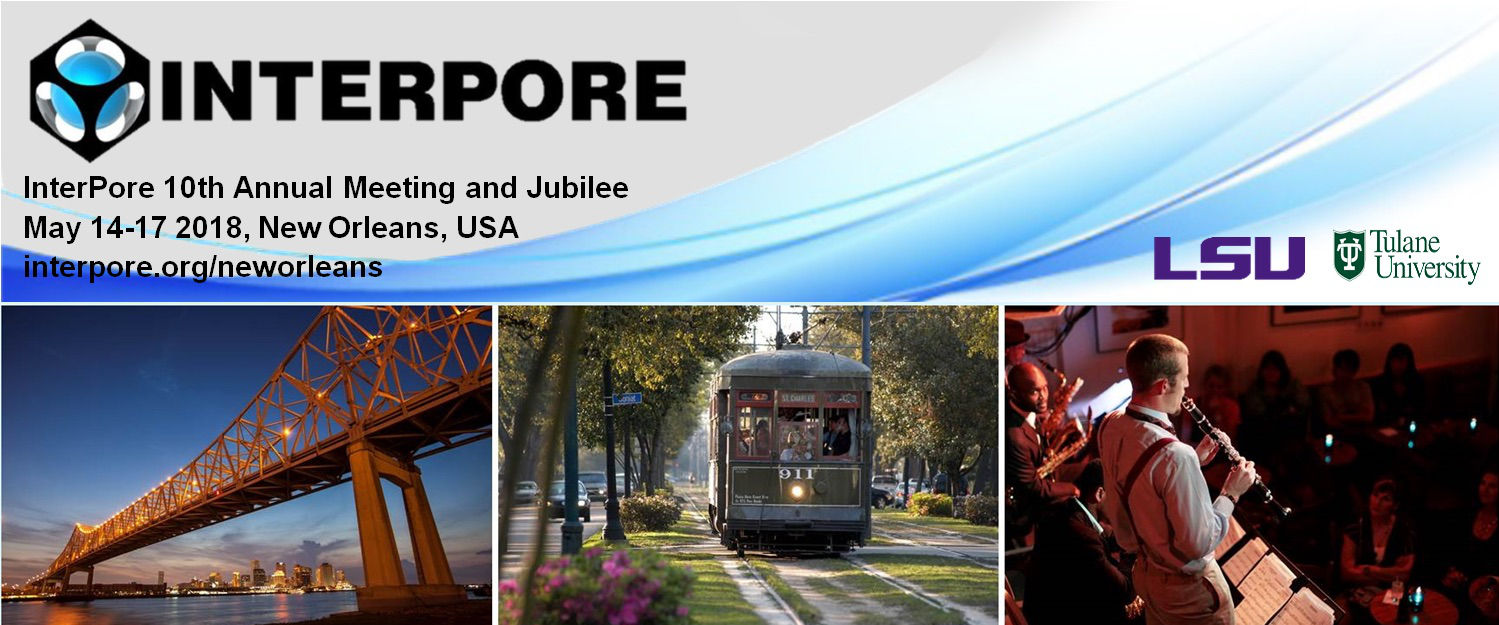#InterPore2018 New Orleans

14-17 May 2018
New Orleans
US/Central timezone

## Advances in the modelling of void clusters and transient wettability

15 May 2018, 10:26
15m
New Orleans

#### New Orleans

Oral 20 Minutes MS 2.23: Network Models

### Speaker

Prof. GPeter Matthews (University of Plymouth)

### Description

We present a major new advance in the interpretation of percolation characteristics, provided by mercury porosimetry, porometry, water retention for soils, and, for nanoscale porosity, Grand Canonical Monte Carlo simulations matched to surface area adsorption measurements. The new method provides a complete analysis of all the void types, providing the user with cumulative distributions that asymptote with size towards the accessible sample porosity. It employs a quasi-Bayesian inverse modelling approach that interprets the percolation characteristic in terms of pores, pore-throats and void clusters, and is shown to be much more accurate than the traditional but incorrect distributions based on the slope of the percolation intrusion characteristic. The advances are applicable to a wide range of micro and mesoporous non-ordered porous materials. Their utility is illustrated by application to three porous materials of current interest: (i) graphite manufactured in Japan for the cores of next generation nuclear reactors, (ii) microporous calcium carbonate being developed for delayed oral drug and flavour delivery, and (iii) naturally occurring hydrophobic soil which will become less fertile and more prone to causing floods under expected climate change conditions. We also describe an improved wetting algorithm, of use not only for soil but also for other important systems such as membranes and fuel cells.

The new void analysis method uses a Boltzmann-annealed amoeboid simplex to inverse model the percolation characteristic. The convergence of the simulated onto the experimental percolation characteristic is automated within the PoreXpert(R) software package [Levy et al. (2015)]. The resulting void structure comprises a unit cell' with periodic boundary conditions, containing up to 108$\,$000 void features. Since the solution to the inverse problem is non-unique, a series of stochastic solutions is generated, and the most statistically representative structure is chosen. For the graphite, a size-range gap between the derived GCMC and direct mercury intrusion percolation data is reflected in a wider range of stochastic generations due to the uncertainty for voids of around 1$\mu$m [Jones et al, 2018]. The percolation characteristic of the representative structure is then forward modelled. A comparison of the intrusion pressure of each pore with respect to the applied pressure then demonstrates whether each feature is a true pore or a cluster of voids [Matthews et al. 2017].

The working equation for the wetting algorithm, derived from that of Bosanquet, is:

$\dot{x} = \left[ \frac{b \, \left( 1 - \mathrm{e}^{-a ( t_\mathrm{full} - t_\mathrm{entry})} \right)^2}{2 a \left\lbrace t_\mathrm{full} - t_\mathrm{entry} - (1/a) \left(1 - \mathrm{e}^{-a(t_\mathrm{full} - t_\mathrm{entry})} \right) \right\rbrace } \right] ^{1/2}$

$a$ and $b$ are constants defined as

$a = \frac{8 \eta}{r^2\rho} \, , \, b = \frac{P_e}{\rho} + \frac{2 \gamma \mathrm{cos}\theta}{r\rho}$

$x$ is the distance travelled by the liquid front in time $t$ into a cylinder of radius $r$, $\eta$ and $\rho$ are the dynamic viscosity and density of the wetting liquid, $\rho$ is the liquid density and $P_e$ is the external pressure applied at the entrance of the capillary tube. $\gamma$ is the interfacial tension at the meniscus, and $\theta$ the contact angle of the intruding fluid with the solid surface. $t_\mathrm{entry}$is the time when liquid enters a particular pore-throat and $t_\mathrm{full}$ when it becomes completely full. The progress of the many thousands of simultaneous wetting events are found by use of a forward Euler method, which reduces the calculation time from weeks to tens of hours. For the soil, the wetting throughout the network stabilises at a timestep of 10 microseconds, which also then equates to the so-called Haines jumps' of the wetting front.
With respect to the three materials we answer three specific questions: (i) how does the void structure of the nuclear graphite differ if the manufacturing process is changed to increase the density, (ii) can additional microporosity be generated by packing the microporous calcium carbonate particles, and (iii) how do molecular scale hydrophobicity transitions upscale to cause soil hydrophobicity at core and field scale?

### References

Bosanquet CH, (1923) Philosophical Magazine, 6, 525-531
Jones KL, Laudone GM and Matthews GP (2018) Carbon, 128, 1-11.
Levy CL, Matthews GP, Laudone GM, Gribble CM et al. (2015) Industrial and Engineering Chemistry Research, 54, 9938-9947
Matthews GP, Levy CL, Laudone GM, Jones KL et al. (2017) Transport in Porous Media, submitted for publication.